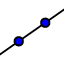# Point, Line, Plane Naming

Undefined terms: A point is a location and is named by a capital letter. A line is made up of points and has no thickness or width. It is named with two letters representing points on the line, or a lowercase script letter. A plane is an infinite flat surface made up of points and is named by a capital script letter or by three letters representing non collinear points on a plane. Collinear points can be contained on a line. Coplanar points can be contained on a plane.
FIRST DIAGRAM: Drag the diagram around by moving the points (you can even make it spin!).

1. Name 3 collinear points. (Turn the diagram if needed)

2. Name 3 noncollinear points:

3. Name a plane.

4. Name in a different way. (Choose all that apply)

Select all that apply
• A
• B
• C
• D
5. Draw , () and then drag it. Notice how the part of the line that is behind the plane is dashed. Draw plane CEA (), and then drag it. Then delete the line and plane that you drew.

6. If possible, draw a line through E and C. Are E and C collinear?

Select all that apply
• A
• B

7. If possible, draw a line through E, G, and C. Are E, G, and C collinear?

Select all that apply
• A
• B

8. If possible, name two points that are non collinear, because you CANNOT draw a line through them.

9. If possible, draw a plane through D, B, and F. Are D, B, and F coplanar?

10. If possible, draw a plane through A, G, E, and B. Move the diagram around to see if the four points are on the plane. Are A, G, E, and B coplanar?

11. If possible, name 3 points that are NOT coplanar, because you CANNOT draw a plane through them.

SECOND DIAGRAM: Use points F, D, and E to drag the rectangular prism around. Then answer the following questions.

12. Name the intersection of and .

13. How many planes are in the image?

15. Name two planes that do not intersect.